# Solution assignment 05 Unit circle and simple formulas

### Assignment 5

Show using the unit circle: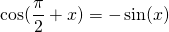### Solution

Draw in the unit circle the angleand next the angle: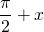The projection on the horizontal-as of point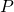corresponding to the first angle and the projection on the vertical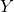-axis of point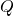corresponding to the second angle are equally large though opposite in sign (which can be shown by congruence).

0